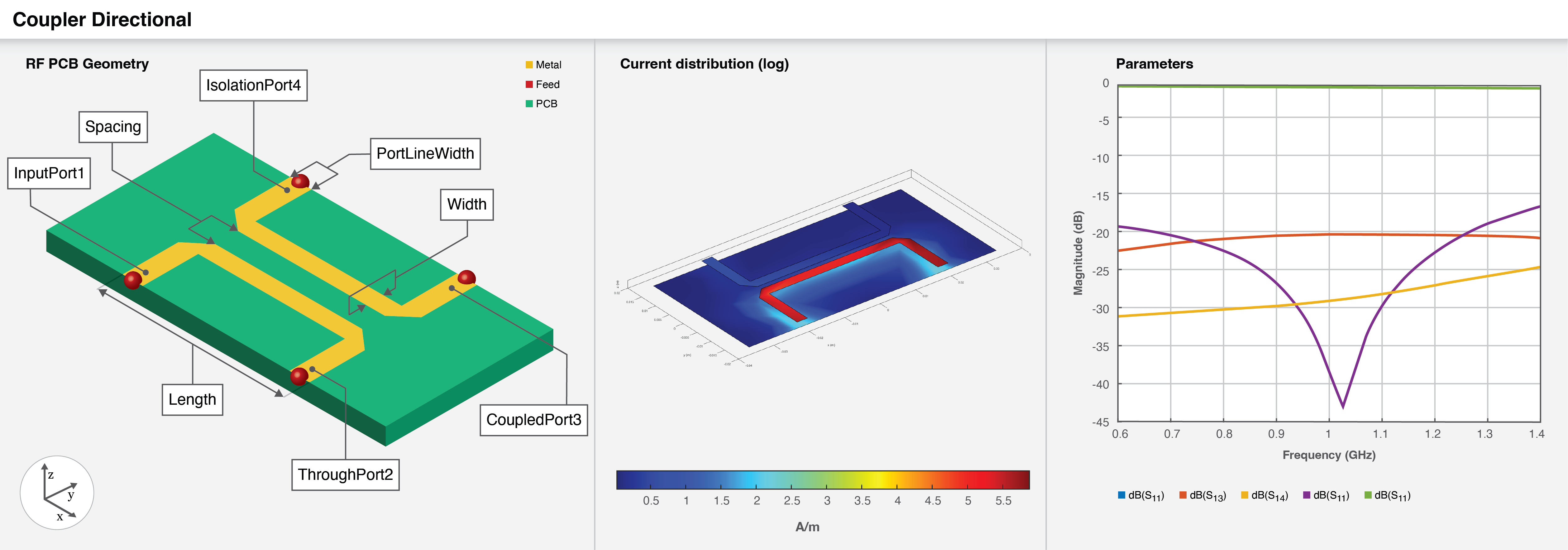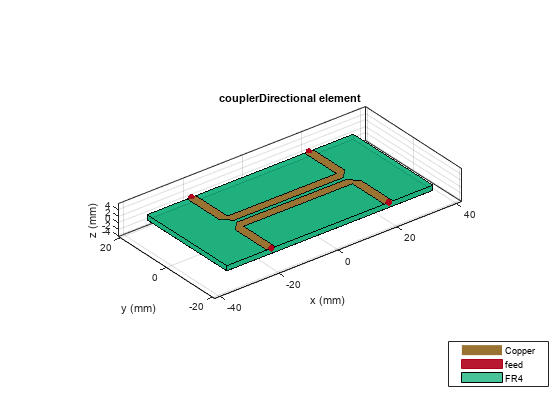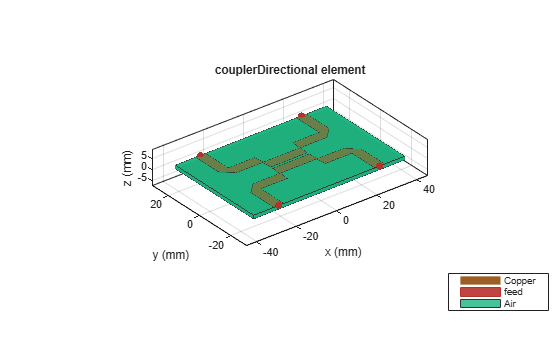# couplerDirectional

Create single section or multi-section directional coupler in microstrip form

## Description

Use the `couplerDirectional` object to create a single section or a multi-section coupler in the microstrip form.## Creation

### Syntax

``coupler = couplerDirectional``
``coupler = couplerDirectional(Name=Value)``

### Description

example

````coupler = couplerDirectional` creates a single section coupler in the microstrip form The default property values are for a resonating frequency of 1 GHz for a 21 dB coupling.```
````coupler = couplerDirectional(Name=Value)` sets Properties using one or more name-value arguments. For example, `couplerDirectional(PortLineWidth=0.0286)` creates a directional coupler with a port line width of 0.0286 meters. Properties not specified retain their default values.```

## Properties

expand all

Length of the coupled line in each section, specified as a positive scalar.

Example: `coupler = couplerDirectional(Length=0.0096)`

Data Types: `double`

Width of the coupled line in each section, specified as a positive scalar or vector.

Example: `coupler = couplerDirectional(Width=0.0036)`

Data Types: `double`

Spacing between the coupled lines in each section, specified as a positive scalar or vector.

Example: `coupler = couplerDirectional(Spacing=0.0016)`

Data Types: `double`

Number of coupled line sections, specified as a positive scalar. To create a multi-section directional coupler, the number of sections must be greater than one.

Example: `coupler = couplerDirectional(NumSections=2)`

Data Types: `double`

Bend type at the corners of the coupler for extending the ports, specified as `'Mitered'`, `'Curved'`, or `'RightAngle'`.

Example: `coupler = couplerDirectional(Corner='Curved')`

Data Types: `char`

Height of the directional coupler from the ground plane in meters, specified as a positive scalar.

In the case of a multilayer substrate, you can use the `Height` property to create a branch line coupler line where the two dielectrics interface.

Example: `coupler = couplerDirectional(Height=0.0026)`

Data Types: `double`

Width of the port line in meters, specified as a positive scalar.

Example: `coupler = couplerDirectional(PortLineWidth=0.0070)`

Data Types: `double`

Length of the ground plane in meters, specified as a positive scalar.

Example: `coupler = couplerDirectional(GroundPlaneLength=0.046)`

Example: double

Width of the ground plane in meters, specified as a positive scalar.

Example: `coupler = couplerDirectional(GroundPlaneWidth=0.046)`

Example: double

Type of dielectric material used as a substrate, specified as a `dielectric` object.

Example: `d = dielectric("FR4");` `coupler = couplerDirectional(Substrate=d)`

Type of metal used in the conducting layers, specified as a `metal` object.

Example: `m = metal("PEC");` `coupler = couplerDirectional(Conductor=m)`

## Object Functions

 `charge` Calculate and plot charge distribution `coupling` Calculate coupling factor of coupler `current` Calculate and plot current distribution `directivity` Calculate directivity of coupler `feedCurrent` Calculate current at feed port `getZ0` Calculate characteristic impedance of transmission line `isolation` Calculate isolation of coupler `layout` Plot all metal layers and board shape `mesh` Change and view mesh properties of metal or dielectric in PCB component `shapes` Extract all metal layer shapes of PCB component `show` Display PCB component structure or PCB shape `sparameters` Calculate S-parameters for RF PCB objects

## Examples

collapse all

Create a default directional coupler.

`coupler = couplerDirectional`
```coupler = couplerDirectional with properties: Length: 0.0397 Width: 0.0028 Spacing: 0.0013 NumSections: 1 Corner: 'Mitered' Height: 0.0016 PortLineWidth: 0.0029 GroundPlaneLength: 0.0697 GroundPlaneWidth: 0.0345 Substrate: [1x1 dielectric] Conductor: [1x1 metal] ```

View the coupler.

`show(coupler)`Create a three-section symmetrical directional coupler on a Teflon substrate.

``` coupler = couplerDirectional(Length=18.15e-3, Width=[4.942e-3,... 4.813e-3,4.942e-3],Spacing=[10.95e-3,1.295e-3,10.95e-3],... NumSections=3 ,Substrate=dielectric(EpsilonR=2.2,LossTangent=0.005,... Thickness=0.0016),PortLineWidth=4.942e-3,... GroundPlaneLength=75.5e-3,GroundPlaneWidth=50e-3 )```
```coupler = couplerDirectional with properties: Length: 0.0181 Width: [0.0049 0.0048 0.0049] Spacing: [0.0109 0.0013 0.0109] NumSections: 3 Corner: 'Mitered' Height: 0.0016 PortLineWidth: 0.0049 GroundPlaneLength: 0.0755 GroundPlaneWidth: 0.0500 Substrate: [1x1 dielectric] Conductor: [1x1 metal] ```

View the coupler.

`show(coupler)`Pozar, David M. Microwave Engineering. 4th ed. Hoboken, NJ: Wiley, 2012.